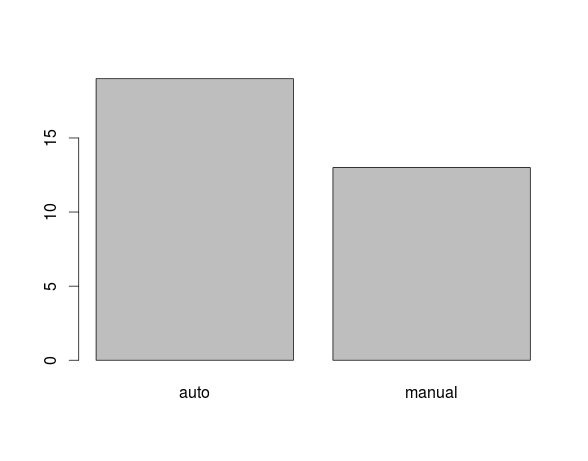# Describing Categorical Data in R

This post will explain how to create tables, calculate proportions, find the mode, and make plots for categorical variables in R. Before providing examples below is the script needed to setup the data that we are using

```cars <- mtcars[c(1,2,9,10)]
cars\$am <- factor(cars\$am, labels=c('auto', 'manual'))
cars\$gear <- ordered(cars\$gear)```

Tables

Frequency tables are useful for summarizing data that has a limited number of values. It represents how often a particular value appears in a dataset. Forexample, in our cars dataset, we may want to know how many different kinds of transmission we have. To determine this, use the code below.

```> transmission_table <- table(cars\$am)
> transmission_table

auto manual
19     13```

Here is what we did.

1. We created the variable ‘transmission_table’
2. In this variable, we used the ‘table’ function which took information from the ‘am’ variable from the ‘cars’ dataset.
3. Final we displayed the information by typing ‘transmission_table’ and pressing enter

Proportion

Proportions can also be calculated. A proportion will tell you what percentage of the data belongs to a particular category. Below are the proportions of automatic and manual transmissions in the ‘cats’ dataset.

```> transmission_table/sum(transmission_table)

auto  manual
0.59375 0.40625```

The table above indicates that about 59% of the sample consists of automatic transmissions while about 40% are manual transmissions

Mode

When dealing with categorical variables there is not mean or median. However, it is still possible to calculate the mode, which is the most common value found. Below is the code.

```> mode_transmission <-transmission_table ==max(transmission_table)
> names(transmission_table) [mode_transmission]
 "auto"```

Here is what we did.

1. We created the variable ‘mode_transmission’ and use the ‘max’ function to calculate the max number of counts in the transmission_table.
2. Next we calculated the names found in the ‘transmission_table’ but we subsetted the ‘modes_transmission variable
3. The most common value was ‘auto’ or automatic tradition,

Plots

Plots are one of the funniest capabilities in R. For now, we will only show you how to plot the data that we have been using. What is seen here is only the simplest and basic use of plots in R and there is a much more to it than this. Below is the code for plotting the number of transmission by type in R.

`> plot(cars\$am)`

If you did this correctly you should see the following.All we did was have R create a visual of the number of auto and manual transmissions.Naturally, you can make plots with continuous variables as well.

Conclusion

This post provided some basic information on various task can be accomplished in R for assessing categorical data. These skills will help researchers to take a sea of data and find simple ways to summarize all of the information.